LEGACY CONTENT. If you are looking for Voteview.com, PLEASE CLICK HERE

This site is an archived version of Voteview.com archived from University of Georgia on May 23, 2017. This point-in-time capture includes all files publicly linked on Voteview.com at that time. We provide access to this content as a service to ensure that past users of Voteview.com have access to historical files. This content will remain online until at least January 1st, 2018. UCLA provides no warranty or guarantee of access to these files.

Probability and Statistics                      Name__________________________
Spring 1997 Flex-Mode 45-733
Final Exam
Keith Poole

(10 Points)

1. GSIA and Pitt students independently take an identical Prob-Stat I final examination. We take a sample 5 Pitt students and 7 GSIA students. The mean score of the 5 Pitt students is 82 points with a standard deviation of 15 points and the mean score of the 7 GSIA students is 88 with a standard deviation of 19 points. Assume that the scores from both schools are normally distributed. Construct 99 percent confidence limits for the difference in the true mean scores.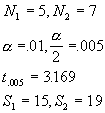Small sample interval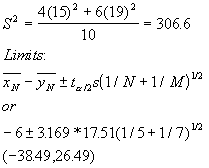Probability and Statistics                      Name__________________________
Spring 1997 Flex-Mode 45-733
Final Exam
Keith Poole

(10 Points)

2. Suppose the arrival of customers at a hot dog stand is a Poisson process with an average of 10 customers every 15 minutes. What is the probability that at least 16 but not more than 24 customers arrive in a period of 30 minutes.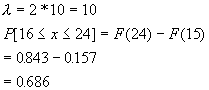Probability and Statistics                      Name__________________________
Spring 1997 Flex-Mode 45-733
Final Exam
Keith Poole

(10 Points)

3. Suppose you draw a sample of 10 first year students and measure their heights and obtain the values (in millimeters): 170, 195, 183, 180, 149, 183, 165, 170, 150, 160; and you draw a sample of 7 second year students and obtain the heights: 171, 164, 178, 178, 160, 154, 163. Assume that height is normally distributed in both populations. Test the null hypothesis that the variances of the two populations are the same against the alternative hypothesis that the variances are not the same (a = .01).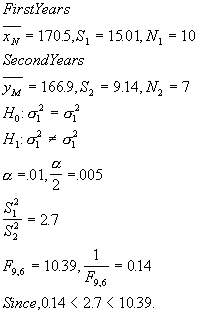DO NOT REJECT.

Probability and Statistics                      Name__________________________
Spring 1997 Flex-Mode 45-733
Final Exam
Keith Poole

(10 Points)

4. Suppose we have the bivariate continuous probability distribution: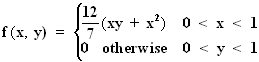Are X, Y independent?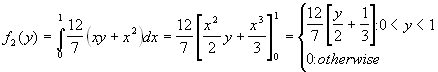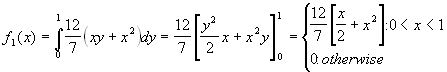Clearly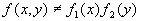Thus NOT INDEPENDENT

Probability and Statistics                      Name__________________________
Spring 1997 Flex-Mode 45-733
Final Exam
Keith Poole

(5 Points)

5. Suppose that the proportion of people in the United States suffering from a rare form of cancer is .0005. We take a random sample of 20,000 people. What is the probability that at least 4 but not more than 14 people in our sample have the cancer?

Poisson Approximation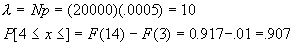Probability and Statistics                      Name__________________________
Spring 1997 Flex-Mode 45-733
Final Exam
Keith Poole

(10 Points)

6. A certain electronic system contains 15 components. Suppose that the probability that any individual component will fail is 0.10 and the components fail independently of each other. Given that at least four but not more than eight of the components have failed, what is the probability that at least six of the components have failed.

BINOMIAL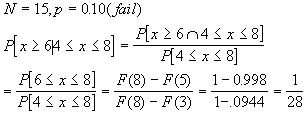Probability and Statistics                      Name__________________________
Spring 1997 Flex-Mode 45-733
Final Exam
Keith Poole

(10 Points)

7. Suppose you draw a random sample of 8 first year masters students and find that their ages are 26, 27, 30, 28, 24, 29, 27, 37, respectively; and you draw a random sample of 6 second year masters students and find that their ages are 39, 23, 24, 28, 29, 29, respectively. Assume age is normally distributed in both populations. Test the null hypothesis that the true population mean ages are the same against the alternative hypothesis that the true means are not the same (a = .01).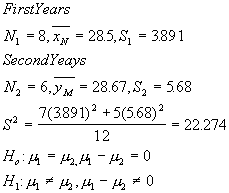Test Statistic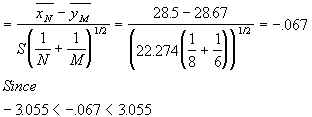DO NOT REJECT

Probability and Statistics                      Name__________________________
Spring 1997 Flex-Mode 45-733
Final Exam
Keith Poole

(10 Points)

8. The times that a cashier at the squirrel hill Giant Eagle spends totaling each person�s groceries are independent variables with a mean of 3.1 minutes and a standard deviation of 2.5 minutes. What is the approximate probability that it will take less than 5 hours to process the purchases of 100 customers?

CLT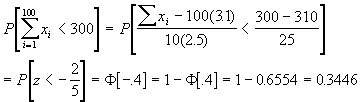Probability and Statistics                      Name__________________________
Spring 1997 Flex-Mode 45-733
Final Exam
Keith Poole

(10 Points)

9. Suppose we know that weight in a large population of men is normally distributed. We take a random sample of 10 men from this population and obtain the weights (in pounds): 281, 176, 190, 169, 205, 185, 199, 218, 177, 200. Compute 95% and 99% confidence limits for the true weight, m .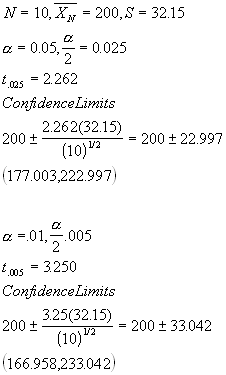Probability and Statistics                      Name__________________________
Spring 1997 Flex-Mode 45-733
Final Exam
Keith Poole

(10 Points)

10. A manufacturer of bolts claims that her manufacturing process is very accurate and that the mean length of a certain type of bolt is 20cm with a standard deviation of .1cm. You take a random sample of 29 bolts and obtain a sample standard deviation of .15cm. Assume that the length of the bolts is normally distributed. Test the null hypothesis that the true variance is 0.01 against the alternative hypothesis that the true variance is not equal to 0.01. Do you accept or reject her claim? Use a = .10.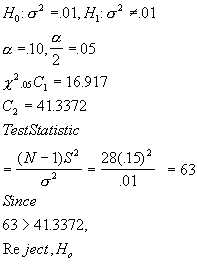Probability and Statistics                      Name__________________________
Spring 1997 Flex-Mode 45-733
Final Exam
Keith Poole

(10 Points)

11. Suppose we have the continuous probability distribution: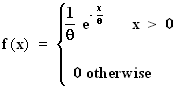Find the maximum likelihood estimator for q.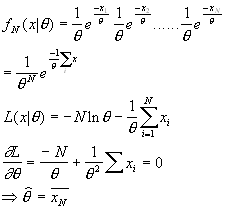Probability and Statistics                      Name__________________________
Spring 1997 Flex-Mode 45-733
Final Exam
Keith Poole

(10 Points)

12. Suppose that we have the bivariate continuous probability distribution: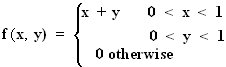Find VAR(2X - 3Y +100).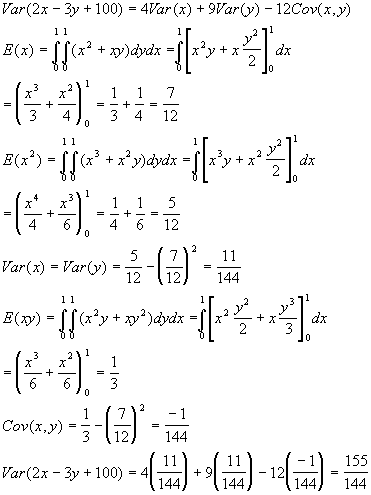Probability and Statistics                      Name__________________________
Spring 1997 Flex-Mode 45-733
Final Exam
Keith Poole

(15 Points)

13. Suppose we have three urns. Urn I contains 3 white, 3 green, and 4 red balls, urn II contains 4 white, 3 green, and 3 red balls, and urn III contains 2 white, 2 green, and 6 red balls. We draw one ball randomly from each urn. If the three selected balls are all the same color, what is the probability that the three balls are green?

P[same color] = P[WWW] + P[GGG] + P[RRR]

=(.3)(.2)(.4) + (.3)(.3)(.2) + (.4)(.3)(.6)

=.114

P[GGG | same color] = P[GGG]/P[same color]

=.018/.114 =18/114

Probability and Statistics                      Name__________________________
Spring 1997 Flex-Mode 45-733
Final Exam
Keith Poole

(10 Points)

14. You own property near an area where oil has been discovered. Suppose the probability that oil is found when a well is drilled in this area is .4. It costs \$1,000,000.00 to drill a well and you only have \$4,000,000.00 that you can spend on the project. You hire an oil drilling firm to drill for oil on your land. What is the probability that you find oil and have money left over?

The only way you could have money left over is if oil is discovered on the 1st , 2nd or 3rd wells.

1st well = 0.4

2nd well = 0.6*0.4=0.24

3rd well = 0.6*0.6*0.6=0.144

Total=0.784

Probability and Statistics                      Name__________________________
Spring 1997 Flex-Mode 45-733
Final Exam
Keith Poole

(10 Points)

15. A large shipment of capacitors is delivered to our manufacturing plant. We take a random sample of 75 capacitors and find that 5 are defective. Compute 95% and 99% confidence limits for p, the true proportion of defective capacitors.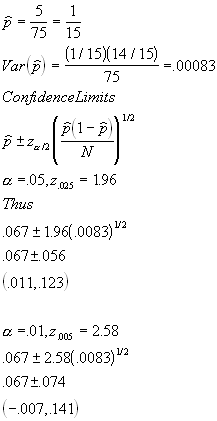Probability and Statistics                      Name__________________________
Spring 1997 Flex-Mode 45-733
Final Exam
Keith Poole

(10 Points)

16. Suppose we have two urns. In urn I there is a single red ball and in urn II there is a single green ball. We select an urn randomly and we add a red ball to the urn. We now randomly select a ball from this urn. If the ball we select is red, what is the probability that the remaining ball in the urn is also red.

This is a twist on the 3 chest problem. There are two possible combinations (RR) or (RW).

3 ways to get the second ball, 2 of which are red.

Thus 2/3